## Sunday 1 November 2015

### SQA Higher Mathematics Examination 2015 (Part One)

This past year, the Scottish Qualifications Authority rolled out a new Higher Mathematics Examination, a test many high school students take to demonstrate they have an acceptable grasp of mathematics to be admitted to university. There are similar examinations on other subjects and Scottish universities typically require that you've taken and passed at least three of these Higher Examinations.

What's surprising about the 2015 examination is how poorly students performed on the test. A C-grade pass was awarded to students who scored as little as 34% on the test, and just 60% was need to receive an A-grade. Does someone who gets barely more than a third of the math right really represent the average student's skill level in mathematics? That's kind of a troubling thought.... When I heard about the low passing scores, I thought I'd try my hand at the test to see if it really was especially difficult.

Spoiler: I didn't finish. Read on to find out which question got the better of me.

In this post I review Part 1 of the examination paper and provide worked solutions. This portion of the test consisted of 15 questions, worth a total of 60 marks, to be solved without a calculator and within a 70 minute time limit. It means you need to know your stuff to finish on time, because you've got only an average of 4 minutes 40 seconds per question, or 1 minute 10 seconds per mark. Sitting at the desk idly thinking about how to solve a particular problem would be a sure way to score poorly on this test.

Problem 1

I thought this was a nice easy question to get the test started. The thing the student needed to recall here was that the dot product of two perpendicular vectors is equal to zero.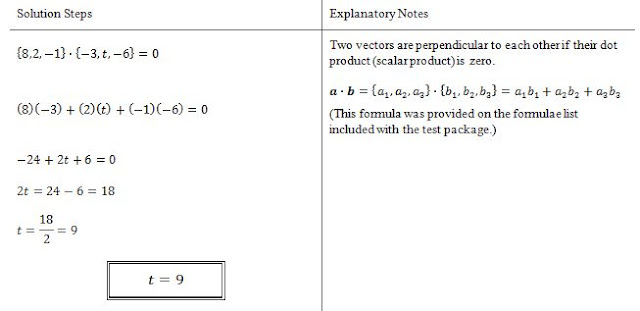Solution to Problem 1

Problem 2

I thought this was also fairly easy. Here the student needed to remember how to define the equation of a line, how to find the derivative of a polynomial, and that the slope of the tangent is equal to the derivative of the function. Taking the derivative of this expression is rudimentary calculus that shouldn't be a problem for most high school students.Solution to Problem 2

Problem 3

This one could cost you precious time if you didn't remember the Factor Theorem. That said, high school students who've just recently finished their math courses and have studied for their qualifications exams ought to have known about the Factor Theorem. Using the Factor Theorem, I solved this in about 3:24. A less efficient way to solve this would have involved polynomial division. I estimated that it would've taken me about 5:40 to solve it that way. At nearly 85 seconds per mark, that's a fair bit slower than the 70 seconds per mark average you need to maintain in order to finish the test. However, I used long division and I'm a little out of practice. I think a high school student who's still got synthetic division fresh in her mind might still be able solve this problem in under 70 seconds per mark.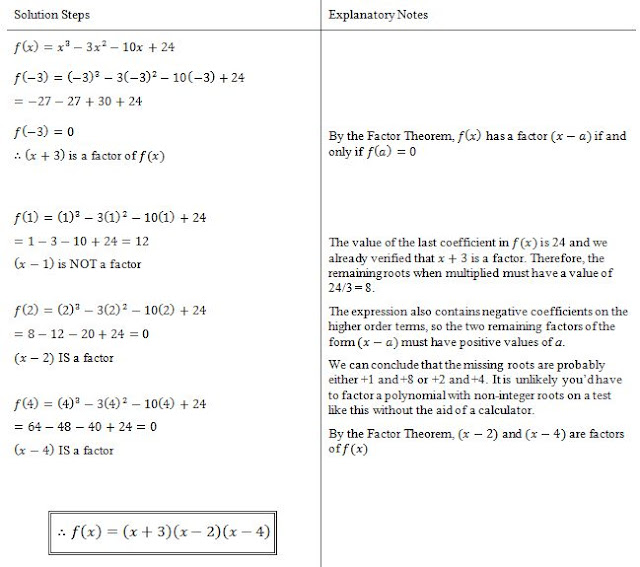Solution to Problem 3

Problem 4

I didn't find this question very difficult either. Here you needed to recognize that the diagram shows a transformation of the cosine function, involving a vertical shift and both vertical and horizontal dilatation. It requires an understanding of how the terms p, q, and r affect the graph.Solution to Problem 4

Problem 5

This was definitely a fluff question. Inverting a linear function should be no problem for someone who just finished their high school mathematics courses.Solution to Problem 5

Problem 6

This question wasn't too difficult, as long as you remembered your laws of logarithms. But don't be deceived by the conciseness of the problem statement. If you didn't recognize which laws to use or how to factorize the numbers inside the log function, you could easily waste some time here.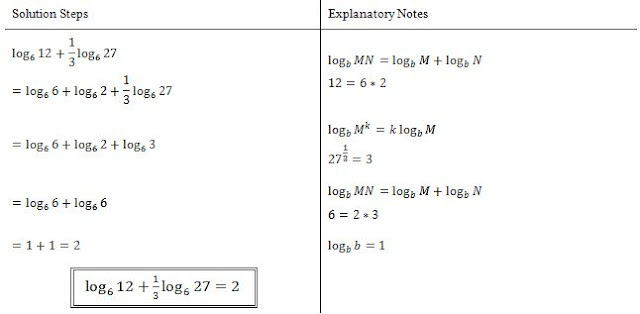Solution to Problem 6

Problem 7

Here's a problem that could really waste some time. The student needed to find the derivative of the function, but in order to solve this quickly it was also necessary to recognize that this function would be a lot easier to differentiate if it was expanded first. If you didn't expand, you'd be putting your high school calculus skills to the test, but also wasting time using the product rule, chain rule, and quotient rule all in this one little problem. I wouldn't be surprised if a significant proportion of students wasted time on this one by just ploughing ahead with chain rules and product rules.Solution to Problem 7

Problem 8
 The algebra on this one is pretty easy, but I can see how a student could easily lose a few marks here. First, you have to recall that the area of a rectangle is length multiplied by width. If you're thinking "duh, that's obvious", well, you'd be surprised how many people have already forgotten this formula or confuse it with something else (say formula for perimeter). Second, you have to define an inequality. Only part of the information you need is given to you (area less than 15 sq. cm). The student would also need to show they're bright enough to limit the length and/or area to positive values (because a rectangle with zero or negative side-length makes no physical sense). It seems like common sense, but it'd be easy for a student to miss that bit while he or she is rushing to finish the test. In which case the student goes ahead and comes up with only half of the answer.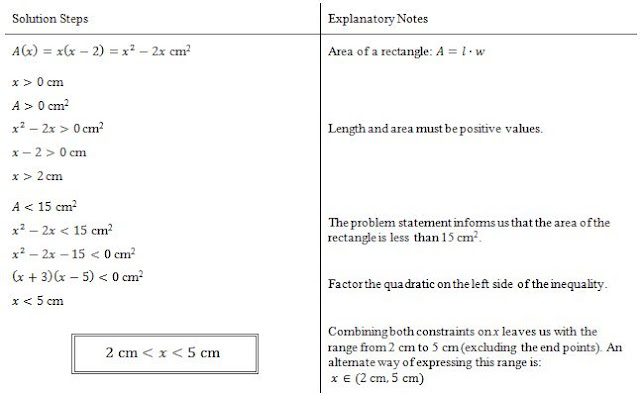Solution to Problem 8

Problem 9

This one could cause a few hangups for students. The question asks if three points lie on the same line, but doesn't give nearly enough information to plot these points on a line or prove that they lie on the same line. That's the sort of thing that can stick in a student's brain as they waste time agonizing over how to prove these points are collinear. The trick here is that you do have enough information to do a test that could prove the three points are NOT collinear. If the slope from A to B is not the same as the slope from B to C, you're done, the points can't possibly be on the same line. The slope from A to B is the same as the slope of the line given by the equation in the problem statement. The slope from B to C is a little less straightforward because you'd have to remember your trigonometric ratios (e.g. from the unit circle).Solution to Problem 9

Problem 10

I thought this one was pretty easy, but I can see this potentially being a colossal waste of time for students that didn't recognize how to attack the problem. Students had to remember the 3-4-5 triangle, or at least what "soh cah toa" meant and then put it to use. That's enough for part (a). Part (b) required some algebraic manipulation of a trig identity. Several identities were provided on the formulae list included with the test, so the student would've needed to decide which one would actually help them solve the problem. A bad choice would've led to a fruitless algebra exercise, costing precious time.Solution to Problem 10

Problem 11

In terms of marks, this question was the most important one of the test. You had to find the tangent to the circle. You could have used implicit differentiation here to get the slope of the tangent at T, but I'm not sure that's taught in high schools these days. I solved part (a) without using calculus. If the student did not know how to use implicit differentiation, the student had to recall that any line from the centre of a circle to a point on the circumference is perpendicular to the circle, and that two lines are perpendicular to each other if one slope is equal to the negative reciprocal of the other slope. For part (b) the student had to take the first derivative to find the tangent to the parabola and then solve for the value of p that makes the tangent to the parabola equal to the tangent from part (a).Solution to Problem 11

Problem 12

Based on the symmetry of a harmonic function like y = acos bx, you might recognize that the unshaded area is 2 times the shaded area, and that the unshaded area would be a negative contribution to the integral because it is below the x-axis. If you did that, you'd have this solved in a just a few seconds. But you could also do this the hard way by solving for a and b and then solving the integral. The latter approach could be a big waste of time, though a well-prepared student probably could still solve it in 2 to 3 minutes, which is pretty close to the 70 seconds per mark you need to average to finish the test on time.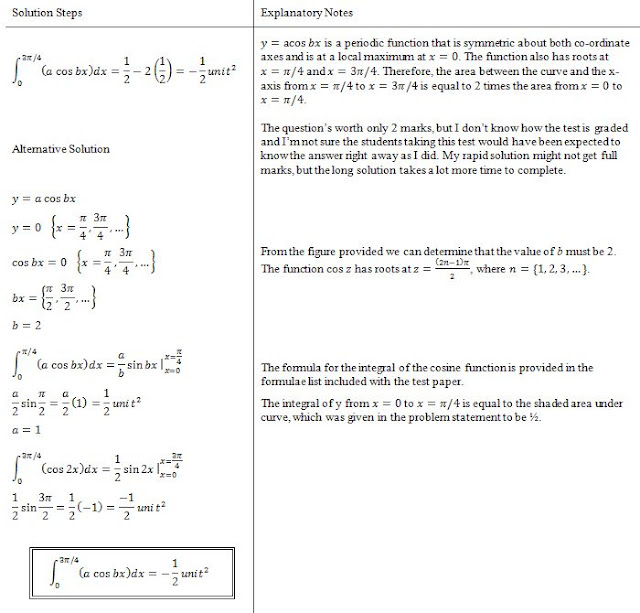Solution to Problem 12

Problem 13

This is the question you were looking for: the problem I didn't solve... within the time allotment. Specifically, it was part (c) that got me. Parts (a) and (b(i)) were no problem. I was confused about what they meant by "images" of P and Q in (b(ii)) but I correctly assumed they meant the points P' and Q' on the inverse function corresponding to points P and Q. I had no idea what they were asking about with Part (c) though so I skipped it. The answer came to me two days later. They were simply asking about function transformations, but they didn't explicitly tell you that in the problem statement. It was merely implied by referring to the images of P, Q, and R. y = 4 - f(x+1) is the transformed version of y = f(x) and the student had to provide the coordinates of the image of point R. Put another way, the student had state where point R would map to after undergoing the same transformations that turn y = f(x) into y = 4 - f(x+1).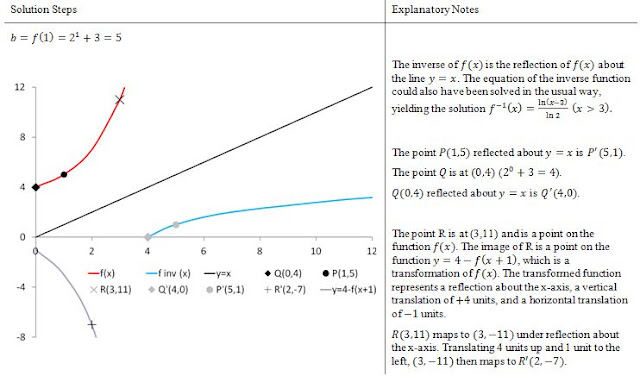Solution to Problem 13

Problem 14

I thought this was an interesting question because you had to think a little bit about how a circle could intersect the axes at exactly three points, and there are two correct answers to this problem. One possibility is that the circle just touches one axis and intersects the other axis at two points. The other possibility is that the circle intersects the axes at the point (0,0) with an additional intersection point on each of the axes. In either case, you start by finding the centre of the circle, which is pretty straightforward because that information can be extracted from the equation. If the circle intersects the origin, set k = 0 and solve for the radius (equal to the distance from the origin to the centre of the circle). If the circle touches one axis but intersects the other at two points, the set the radius to whichever centre co-ordinate has larger magnitude (in this problem it was the x-coordinate), then solve for k.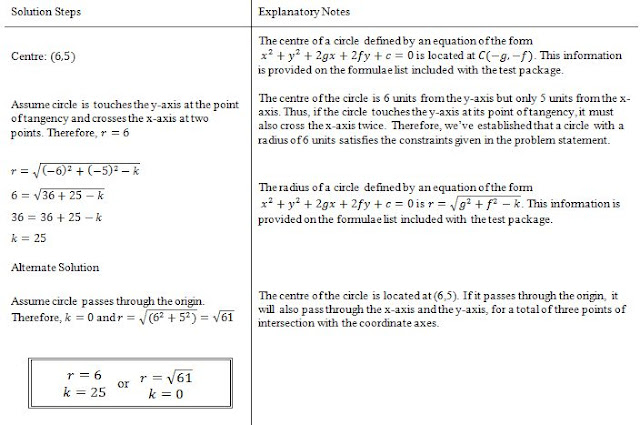Solution to Problem 14

Problem 15Here the student is given an expression for the rate of change of the temperature of some coffee and is asked to find an expression for the temperature versus time. This problem requires an integration. It's a fairly simple integration since it's just a linear function. An important thing to remember here is to include the integration constant and then solve for it using the boundary conditions provided in the problem statement.

 Solution to Problem 15

In summary, Part One of the 2015 SQA Higher Mathematics Examination didn't have any questions that were especially difficult to solve using high school math skills. However, the time limit likely hurt a lot of students. Students needed to quickly recognize an efficient way to solve each problem and work through the solutions with confidence. Excluding 13 (c), I finished with time to spare, though not as much spare time as I expected. On top of the amount of time to read the questions and go through the process of calculating and writing the solutions, students likely had a relatively small cushion of time for any pauses to think about the problems, review solutions, or second-guess themselves. To do really well on this exam you definitely had to know your stuff well and at times demonstrate a little ingenuity to find the fastest route to the answer. These are reasonable expectations for a math exam (after all, if the test was too easy, it'd be difficult to distinguish the most brilliant students from the average). All that being said, because the questions weren't all that difficult, I believe the average student should've at least been able to earn an honest pass with a grade above 50%.

#### 1 comment:

1.DR EMU WHO HELP PEOPLE IN ANY TYPE OF LOTTERY NUMBERS
It is a very hard situation when playing the lottery and never won, or keep winning low fund not up to 100 bucks, i have been a victim of such a tough life, the biggest fund i have ever won was 100 bucks, and i have been playing lottery for almost 12 years now, things suddenly change the moment i came across a secret online, a testimony of a spell caster called dr emu, who help people in any type of lottery numbers, i was not easily convinced, but i decided to give try, now i am a proud lottery winner with the help of dr emu, i won \$1,000.0000.00 and i am making this known to every one out there who have been trying all day to win the lottery, believe me this is the only way to win the lottery.